Coming to Statistica
in 2022/2023

Zoom tutorials
using Explaineverything app
We a record sessions for you to listen to later

CALL STATISTICA NOW

.

E-mail     anne@statistica.com.au

# Place         Willetton, Western Australia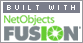# Mathematics & Statistic Tutor Perth - SPSS Help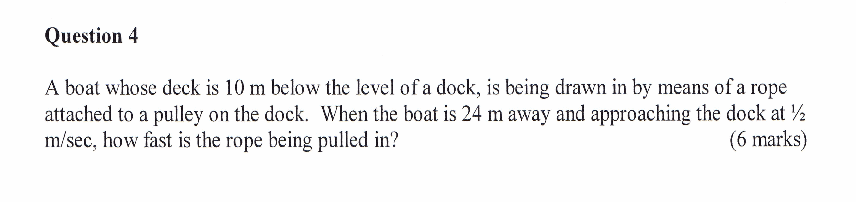# When I say read the question, I mean READ THE QUESTION!!!STEP ONE

Use your pens to act out the movements of the variables, notice which distances increase, decrease and which stay the same.

if the quantity is staying the same then the rate of change is zero
if the quantity is decreasing then the rate of change is negative
if the quantity is increasing then the rate of change is positive

On paper draw a diagram.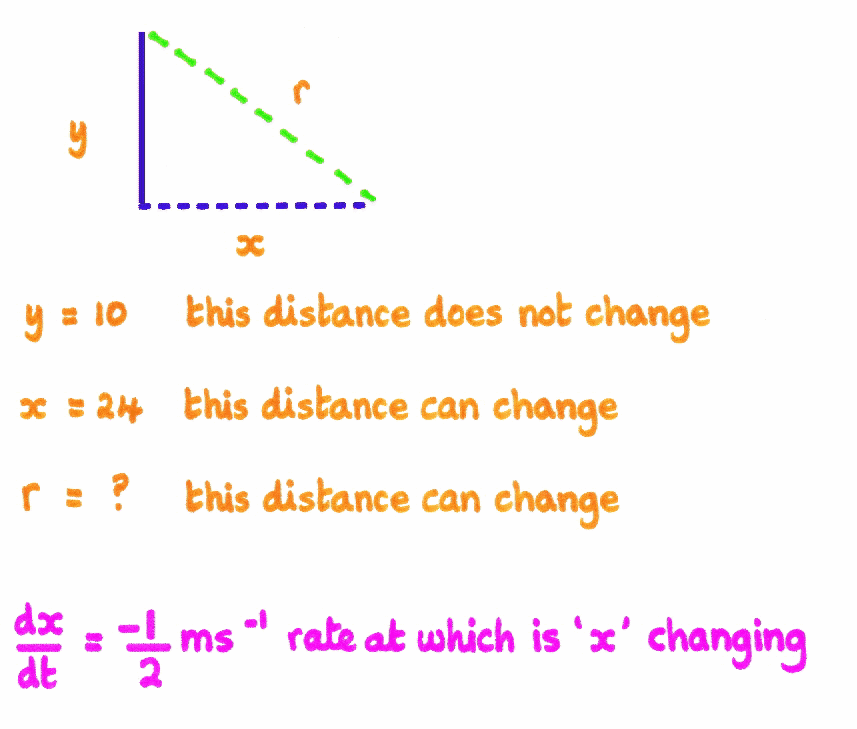STEP TWO Look at the shape and think of any formulae or equations that are associated with the shape. Look at the units in the question eg  ms-1   this will be a length changing       m2s-1 this will be an area changing       m3s-1 this will be a volume changing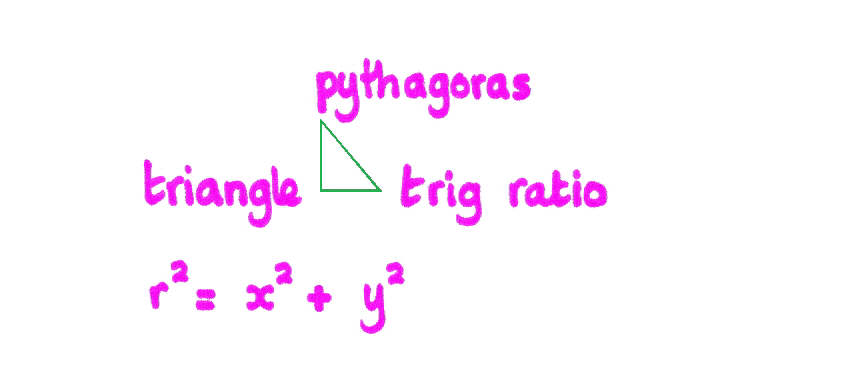STEP THREE Differentiate with respect to time as we want the rate at which something is happening not just a length area or volume.  Look at the variables or letters, unless it is a ‘t’, then we will always multiply by the amount d(letter)/dt if not we are treating the letter as if it were the letter t! Really does ‘r’ look like ‘t’ ?So what do we do? We use the rules of differentiation ie Trigonometric, Logarithm Chain ... but if it is not a ‘t’ then DON’T FORGET TO WE MUTIPLY BY d(letter)/dtThis is now the General Expression of how the variables are changing towards each other.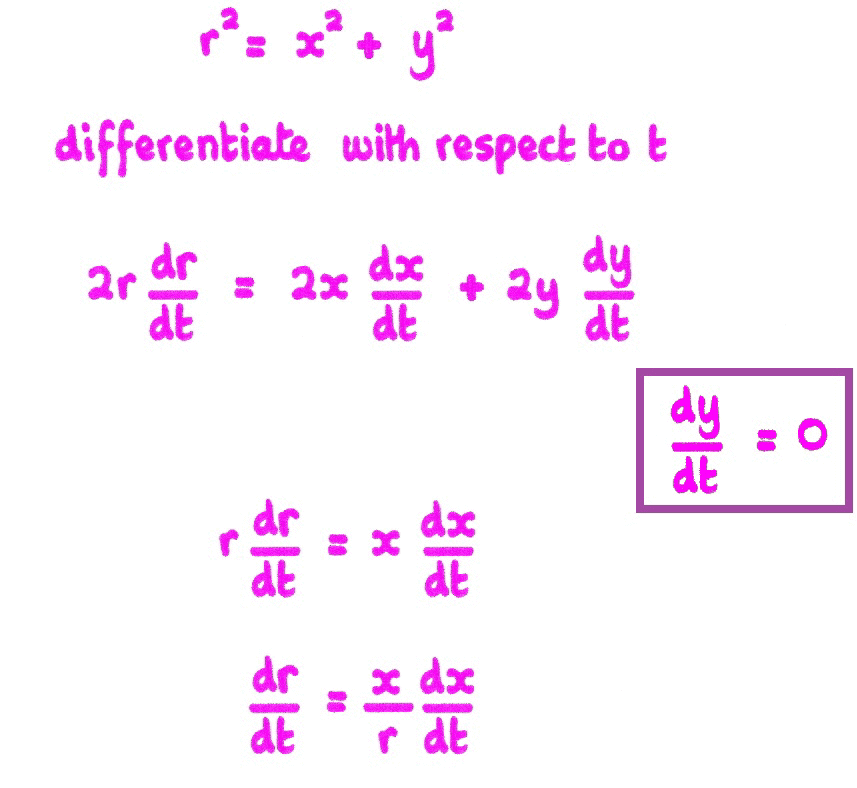STEP FOUR Now we need to gather all the information to find a specific rate of change at some particular value.  We will substitute the given values into the General Expression though we may need to do some more calculations to find the value of other variables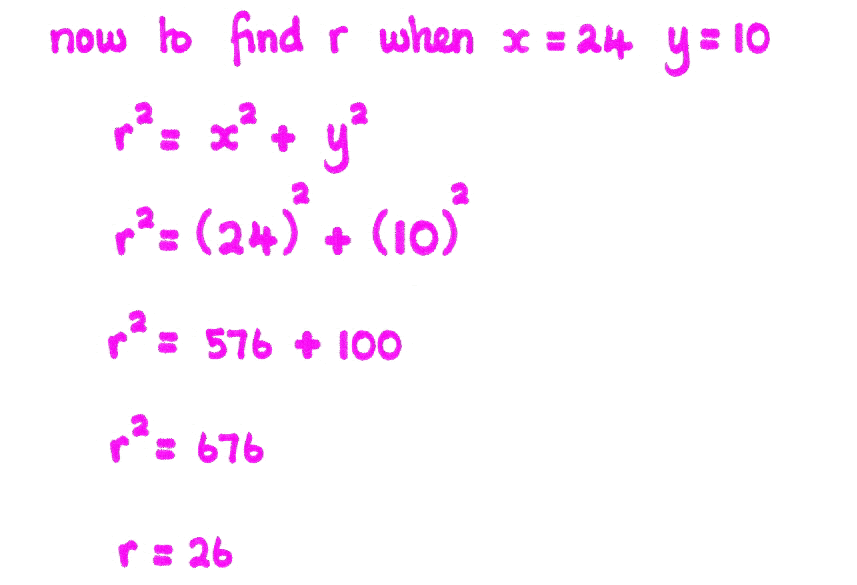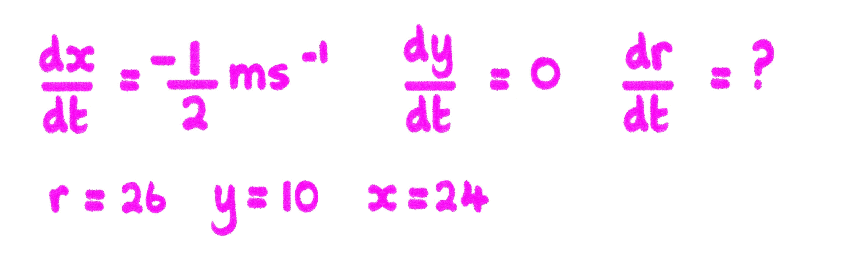STEP FIVE Now answer the question so which rate of change did you want?  Just remember is the quantity changing or not,if the quantity is staying the same then the rate of change is zeroif the quantity is decreasing then the rate of change is negativeif the quantity is increasing then the rate of change is positiveUse your pens to act out the movements of the variables, notice which quantities increase, decrease and which stay the same.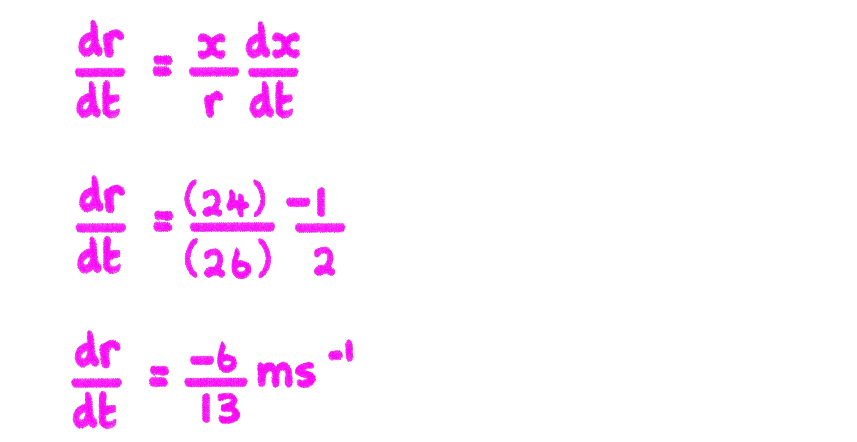[Home] [Parents] [Contact us] [Services] [Mathematics] [Statistics] [School]When you see the frogPress for an explanation